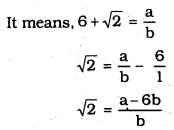# KSEEB SSLC Class 10 Maths Solutions Chapter 8 Real Numbers Ex 8.3

KSEEB SSLC Class 10 Maths Solutions Chapter 8 Real Numbers Ex 8.3 are part of KSEEB SSLC Class 10 Maths Solutions. Here we have given Karnataka SSLC Class 10 Maths Solutions Chapter 8 Real Numbers Exercise 8.3.

## Karnataka SSLC Class 10 Maths Solutions Chapter 8 Real Numbers Exercise 8.3

Question 1.
Prove that $$\sqrt{5}$$ is irrational.
Solution:
Let us assume, to the contrary, that $$\sqrt{5}$$ is rational.
∴ $$\sqrt{5}=\frac{a}{b}$$
∴ b × $$\sqrt{5}$$ = a
By Squaring on both sides,
5b2 = a2 …………. (i)
∴ 5 divides a2.
5 divides a.
∴ We can write a = 5c.
Substituting the value of ‘a’ in eqn. (i),
5b2 = (5c)2 = 25c2
b2 = 5c2
It means 5 divides b2.
∴ 5 divides b.
∴ ‘a’ and ‘b’ have at least 5 as a common factor.
But this contradicts the fact that a’ and ‘b’ are prime numbers.
∴ $$\sqrt{5}$$ is an irrational number.

Question 2.
Prove that $$3+2 \sqrt{5}$$ is irrational.
Solution:
Let us assume that $$3+2 \sqrt{5}$$ is an irrational number.
Here, p, q, ∈ z, q ≠ 0$$\sqrt{5}$$ is rational number.
∵ $$\frac{p-3 q}{2 q}$$ is rational number.
But $$\sqrt{5}$$ is not a rational number.
∴ $$3+2 \sqrt{5}$$ is an irrational number.

Question 3.
Prove that the following are irrationals :
i) $$\frac{1}{\sqrt{2}}$$
ii) $$7 \sqrt{5}$$
iii) $$6+\sqrt{2}$$
Solution:
i) Let $$\frac{1}{\sqrt{2}}$$ is a rational number.
$$\frac{1}{\sqrt{2}}=\frac{\mathrm{p}}{\mathrm{q}}$$
$$\sqrt{2}=\frac{q}{p}$$
By Squaring on both sides,
2 × p2 = q2
2, divides q2.
∴ 2, divides q
∵ q is an even number.
Similarly ‘p’ is an even number.
∴ p and q are even numbers.
∴ Common factor of p and q is 2.
This contradicts the fact that p and q also irrational.
∴ $$\sqrt{2}$$ is an irrational number.
∴ $$\frac{1}{\sqrt{2}}$$ is an irrational number.

ii) Let $$7 \sqrt{5}$$is a rational number.
∴ $$7 \sqrt{5}=\frac{p}{q}$$
$$\sqrt{5}=\frac{p}{7 q}$$
Here,$$\frac{p}{7 q}$$ is one rational number.
It means $$\sqrt{5}$$ which is equal also a rational number.
This contradicts to the fact that $$\sqrt{5}$$ is an irrational number.
This contradicts to the fact that $$7 \sqrt{5}$$ is rational number.
∴ $$7 \sqrt{5}$$ is a rational number.

iii) Let $$6+\sqrt{2}$$ is a rational number.$$\frac{a-6 b}{b}$$ is a rational number, b
∴ $$\sqrt{2}$$ is also rational number.
This contradicts to the fact that $$\sqrt{2}$$ is an irrational number.
This contradicts to the fact that $$6+\sqrt{2}$$ is a rational number.
∴ $$6+\sqrt{2}$$ is an irrational number.

We hope the given KSEEB SSLC Class 10 Maths Solutions Chapter 8 Real Numbers Ex 8.3 will help you. If you have any query regarding Karnataka SSLC Class 10 Maths Solutions Chapter 8 Real Numbers Exercise 8.3, drop a comment below and we will get back to you at the earliest.

error: Content is protected !!